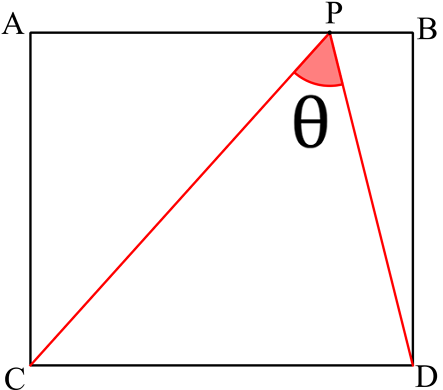# Optimize Peripheral Vision

Geometry Level 3$ABDC$ is a rectangle and $P$ is a point on the straight line $\overline{AB}$. The lengths of $\overline{AC}$ and $\overline{AB}$ are 1 and $\dfrac{2}{\sqrt3}$, respectively. Find the maximum value of $\theta$ to the nearest integer.